Comment on page

# Regularization

Watch this. Also explains about ISO surfaces, lp norm, sparseness.
(what is?) Regularization (in linear regression) - to find the best model we define a loss or cost function that describes how well the model fits the data, and try minimize it. For a complex model that fits even the noise, i.e., over fitted, we penalize it by adding a complexity term that would add BIGGER LOSS for more complex models.
• Bigger lambda -> high complexity models (deg 3) are ruled out, more punishment.
• Smaller lambda -> models with high training error are rules out. I.e., linear model on non linear data?, i.e., deg 1.
• Optimal is in between (deg 2)
Rehearsal on vector normalization - for l1,l2,l3,l4 etc, what is the norm? (absolute value in certain cases)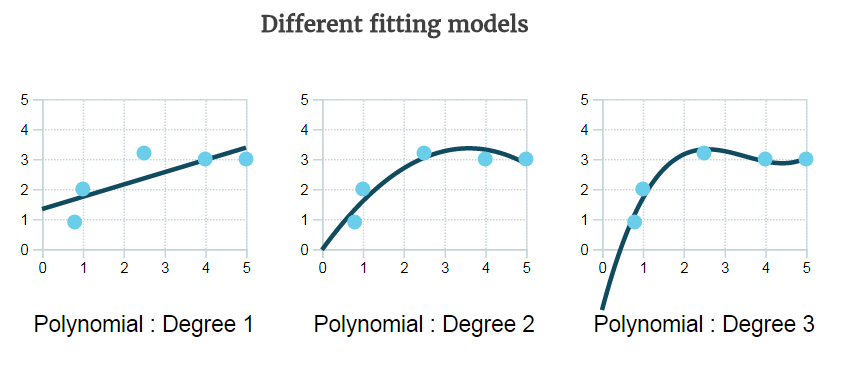(Difference between? And features of) L1 vs L2 as loss function and regularization.
• L1 - moves the regressor faster, feature selection by sparsing coefficients (zeroing them), with sparse algorithms it is computationally efficient, with others no, so use L2.
• L2 - moves slower, doesn't sparse, computationally efficient.
Why does L1 lead to sparity?
• Intuition + some mathematical info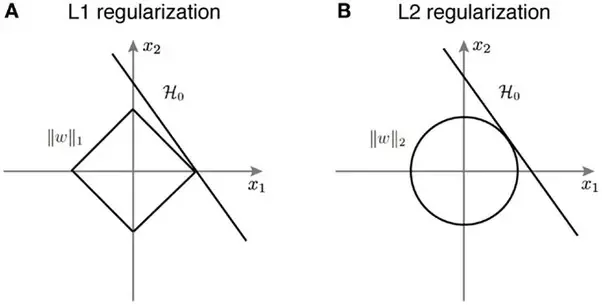• L1 & L2 regularization add constraints to the optimization problem. The curve H0 is the hypothesis. The solution is a set of points where the H0 meets the constraints.
• In L2 the the hypothesis is tangential to the ||w||_2. The point of intersection has both x1 and x2 components. On the other hand, in L1, due to the nature of ||w||_1, the viable solutions are limited to the corners of the axis, i.e., x1. So that the value of x2 = 0. This means that the solution has eliminated the role of x2 leading to sparsity.
• This can be extended to a higher dimensions and you can see why L1 regularization leads to solutions to the optimization problem where many of the variables have value 0.
• In other words, L1 regularization leads to sparsity.
• Also considered feature selection - although with LibSVM the recommendation is to feature select prior to using the SVM and use L2 instead.
• For simplicity, let's just consider the 1-dimensional case.
• L2:
• L2-regularized loss function F(x)=f(x)+λ∥x∥^2 is smooth.
• This means that the optimum is the stationary point (0-derivative point).
• The stationary point of F can get very small when you increase λ, but it will still won't be 0 unless f′(0)=0.
• L1:
• regularized loss function F(x)=f(x)+λ∥x∥ is non-smooth, i.e., a min knee of 0.
• It's not differentiable at 0.
• Optimization theory says that the optimum of a function is either the point with 0-derivative or one of the irregularities (corners, kinks, etc.). So, it's possible that the optimal point of F is 0 even if 0 isn't the stationary point of f.
• In fact, it would be 0 if λ is large enough (stronger regularization effect). Below is a graphical illustration.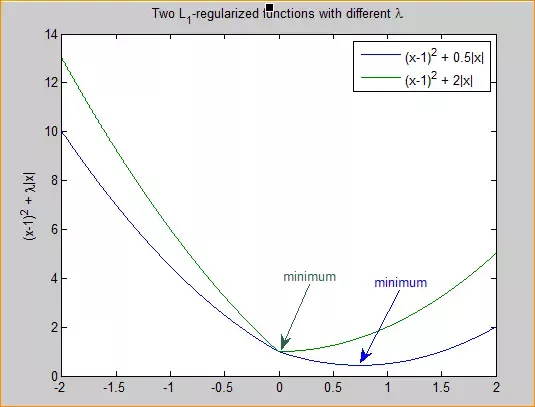In multi-dimensional settings: if a feature is not important, the loss contributed by it is small and hence the (non-differentiable) regularization effect would turn it off.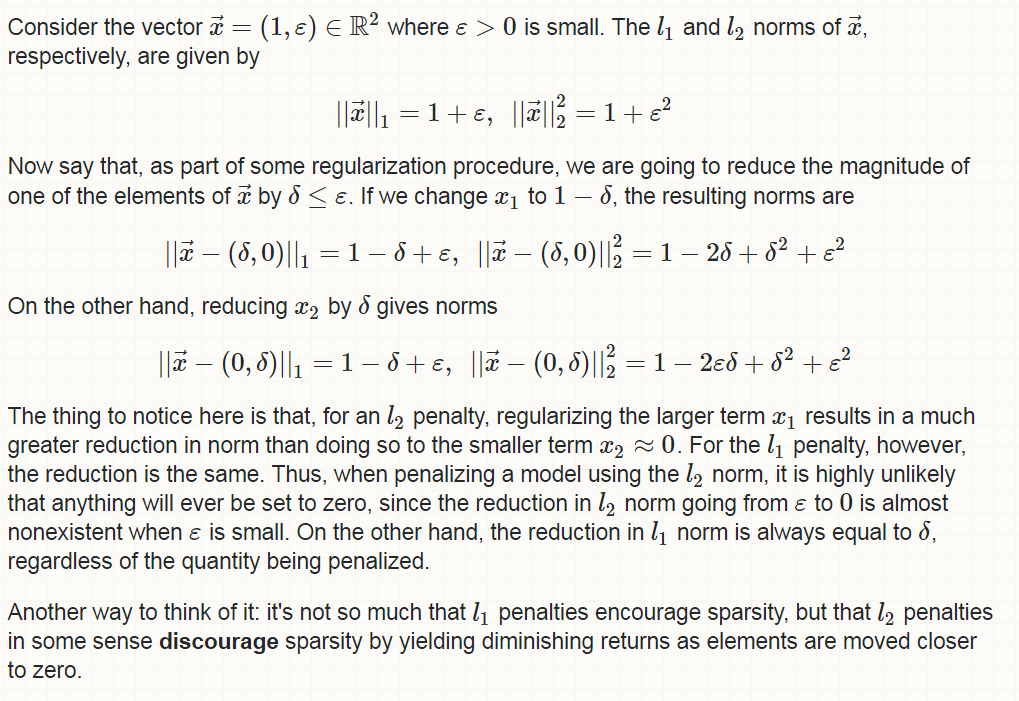(did not watch) but here is andrew ng talks about cost functions.
L2 regularization equivalent to Gaussian prior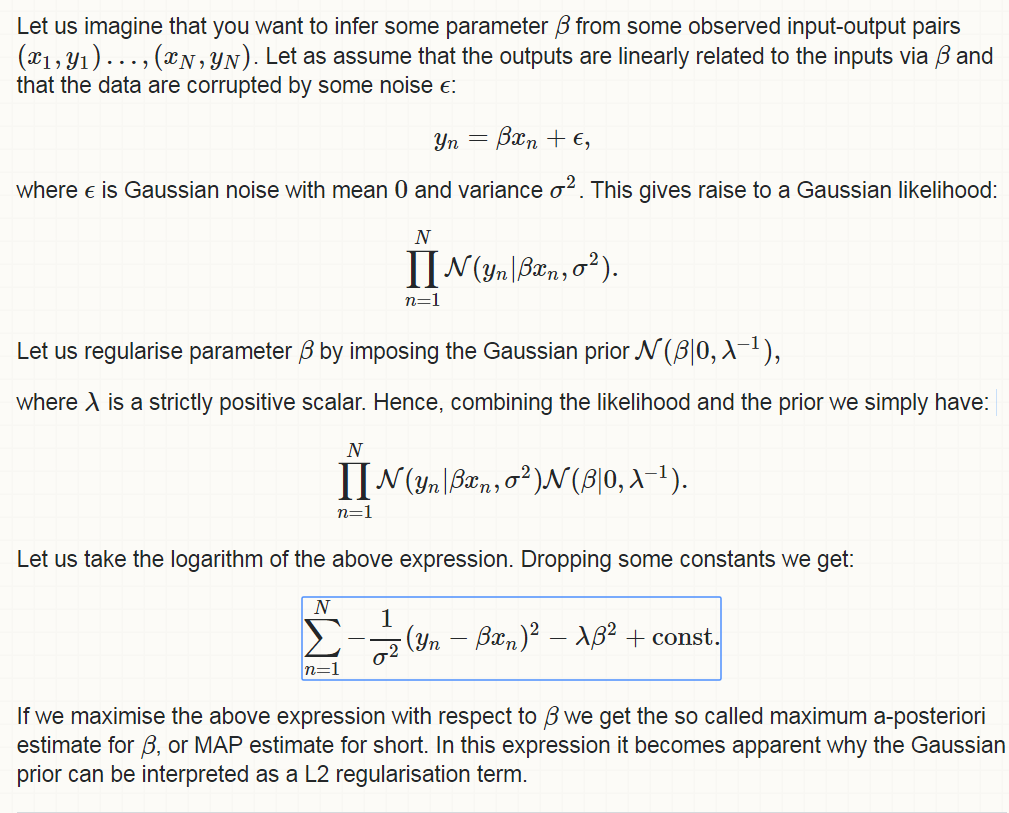L1 regularization equivalent to a Laplacean Prior(same link as above) - “Similarly the relationship between L1 norm and the Laplace prior can be undestood in the same fashion. Take instead of a Gaussian prior, a Laplace prior combine it with your likelihood and take the logarithm.“ How does regularization look like in SVM - controlling ‘C’